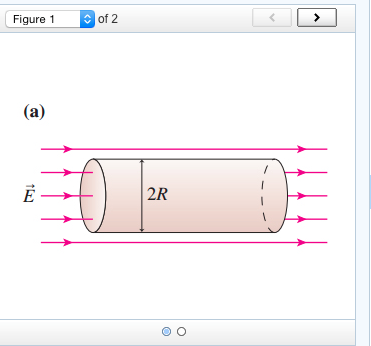# Problem: What is the net electric flux through the cylinder (a) shown in (Figure 1)?Express your answer in terms of the variables E, R, and the constant π.

###### FREE Expert Solution

Electric flux:

$\overline{){{\mathbf{\varphi }}}_{{\mathbf{E}}}{\mathbf{=}}{\mathbf{EAcos\theta }}}$

Electric flux through the left side:

$\begin{array}{rcl}{\mathbf{\varphi }}_{\mathbf{E}\mathbf{1}}& \mathbf{=}& \mathbf{E}\mathbf{\left(}\mathbf{\pi }{\mathbf{R}}^{\mathbf{2}}\mathbf{\right)}\mathbf{c}\mathbf{o}\mathbf{s}\mathbf{180}\mathbf{°}\end{array}$###### Problem Details

What is the net electric flux through the cylinder (a) shown in (Figure 1)?

Express your answer in terms of the variables E, R, and the constant π.